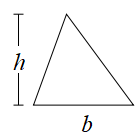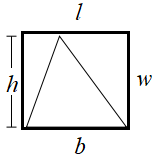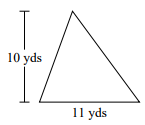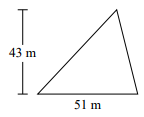# Area of a triangle

Consider any triangle as followsWe can draw a rectangle about the triangle as follows such that its length is b and width is h.We move around triangles to form a parallelogram of which given triangle is exactly half in area. The area of the parallelogram and the rectangle is equal.

So,

Area of triangle = $\frac{1}{2}$ Area of rectangle = $\frac{1}{2}$ l × w = $\frac{1}{2}$ b × h

Find the area of the triangle given below.### Solution

Step 1:

Area of Right Triangle = $\frac{1}{2}$ × b × h; b = base = 11 yd; h = height = 10 yd

Step 2:

Area of Right Triangle = $\frac{1}{2}$ × 11 × 10 = 55 square yd.

Find the area of the triangle given below.### Solution

Step 1:

Area of Right Triangle = $\frac{1}{2}$ × b × h; b = base = 51 m; h = height = 43 m

Step 2:

Area of Right Triangle = $\frac{1}{2}$ × 51 × 43 = 1096.5 square m.